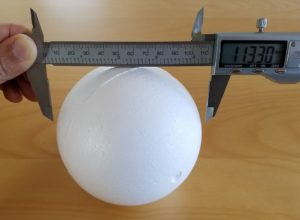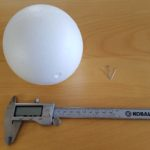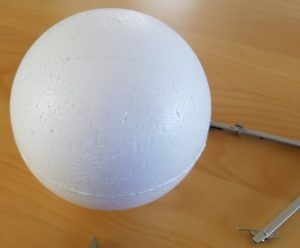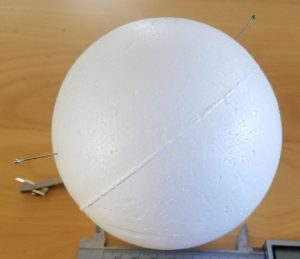24 Jul

I recently purchased a large number of styrofoam balls as supplies for an upcoming build (about which I will post later). The plans for that build required the diameter of the styrofoam balls, to pretty high accuracy. Although the balls were nominally 4 1/2 inches in diameter, I had noticed in a craft shop that their products also had diameters listed in whole numbers of millimeters that were close to the inch ratings, but not precisely equal. Not only could I not find the millimeter diameters listed for this particular size, I needed to know which was closer to reality, the U.S. or the metric measurement. (I had a hunch that given the world-wide nature of manufacturing and the fact that only the U.S. does not use metric, the metric measurements were more likely to be accurate.)Unfortunately, my calipers did not have long enough jaws to clamp down onto the styrofoam balls, as you can see above. So how could I accurately measure the diameter of the sphere? You can’t exactly stick a ruler through the ball, and even if you could, how would you locate two diametrically opposed points? There’s a nice trick with a circle, in which you pick any two points, and then draw a line through one of them perpendicular to the line between them. Where that line intersects the circle is diametrically opposite the other point. (This works because the hypotenuse of any right triangle inscribed in a circle is a diameter.) But is there a three-dimensional version of this?

Fortunately, I was not the first person to face this conundrum; you can find this exact question on Math StackExchange. So first I tried the “accepted” answer: find two planes at exactly right angles with each other (I used a bookshelf, checked for true with a square), shove the sphere into the corner where the planes meet, and measure the distance between the plane and the point of contact to get the radius. Clever, huh?

But in practice, it’s pretty difficult to see exactly where the sphere touches the shelf. As you can see in the photo above, it looks consistent with a 57mm radius but it also looked consistent with a 57.5mm or even a 58mm radius. I wanted to double check with a more accurate method.

Fortunately, there was another answer on that page, involving measuring multiple distances on the surface of the sphere (easy to do with calipers), followed by a rather lengthy and involved calculation. But a little experimentation and figuring arrived at the following method, which is pretty quick and simple, so I thought it deserved its own post.

First,

Materials
sphere to measure
compass
calipers
pinspick a convenient, round distance that appears to be in the neighborhood of a third to a quarter of the way around the sphere; for my styrofoam pieces, I chose 90mm. Using calipers (or a ruler), set the compass to exactly this distance. Choose an arbitrary point on the sphere, and draw a circle with the compass. (You can just see the circle faintly in the picture below.)Now choose another arbitrary point on this circle. We want to find the two points on the circle at that same chosen distance away from this second point. So put the point of the compass on this second point, and draw two small arcs intersecting the circle, one on either side of the chosen point. Carefully measure the straight-line distance between these two points of intersection (not the distance on the surface of the sphere, i.e., use calipers, not a bendy ruler or measuring tape).I stuck a pin into each of the two points as an aid to positioning the jaws of the caliper precisely at those points, as you can see at left. Call that measured distance F; in my case, I got 101.2mm. Call the original chosen distance (the one you drew the circle and arcs with) A. Then the radius of the sphere is ½A√((4A²-F²)/(3A²-F²)). With my numbers, A = 90 and F = 101.2, this came out numerically to 56.495… Hence, I conclude that the actual diameter of the styrofoam balls is 113mm, just slightly below 4 1/2 inches (numerically it comes out to 4.4488 in).

To see exactly why this works and how the formula is derived, check out the StackExchange page. A very pleasant case of the math actually working out to solve a practical problem!